# Machine Learning Basics for Web Application Developers

30 de Aug de 2016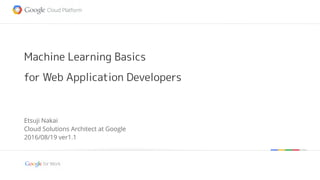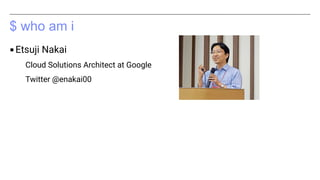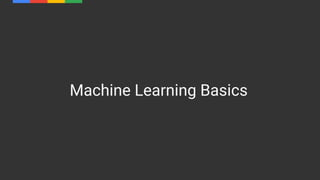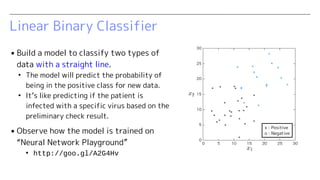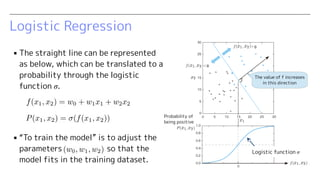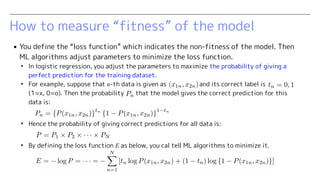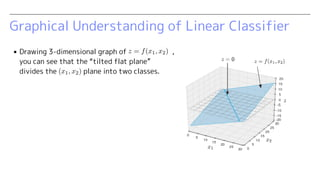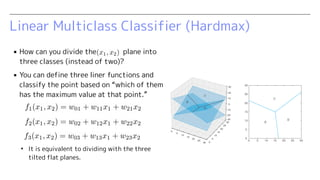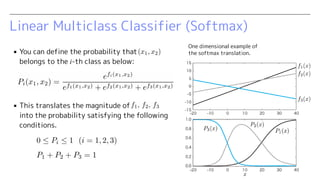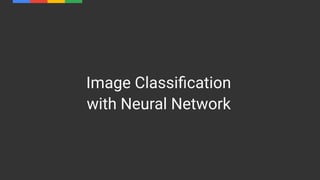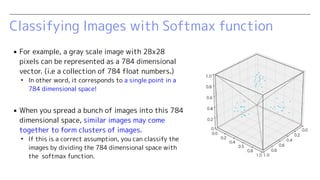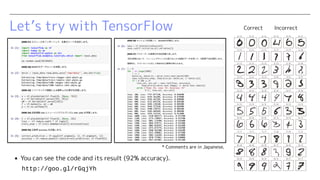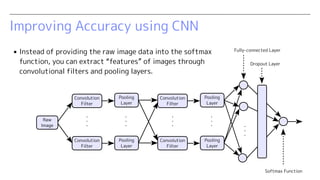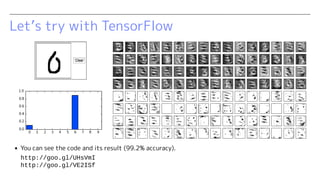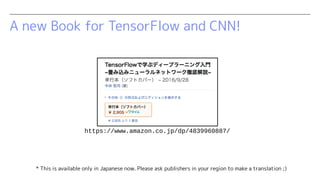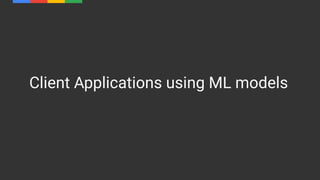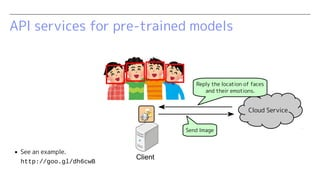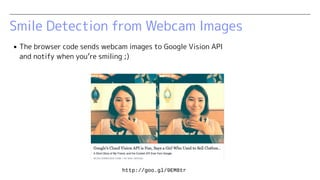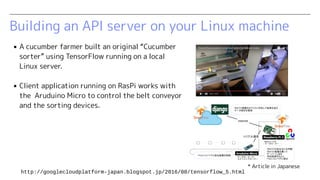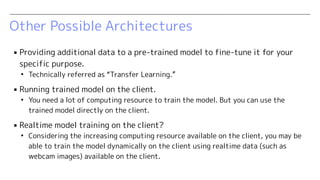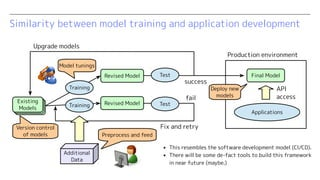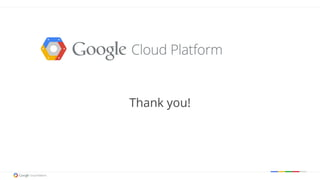1 de 22

### Machine Learning Basics for Web Application Developers

1. Google confidential | Do not distribute Machine Learning Basics for Web Application Developers Etsuji Nakai Cloud Solutions Architect at Google 2016/08/19 ver1.2
2. \$ who am i ▪Etsuji Nakai Cloud Solutions Architect at Google Twitter @enakai00
3. Machine Learning Basics
4. Linear Binary Classifier ▪ Build a model to classify two types of data with a straight line. ● The model will predict the probability of being in the positive class for new data. ● It’s like predicting if the patient is infected with a specific virus based on the preliminary check result. ▪ Observe how the model is trained on “Neural Network Playground” ● http://goo.gl/A2G4Hv x : Positive o : Negative
5. Logistic Regression ▪ The straight line can be represented as below, which can be translated to a probability through the logistic function σ. ▪ “To train the model” is to adjust the parameters so that the model fits in the training dataset. Logistic function σ Probability of being positive The value of f increases in this direction
6. How to measure “fitness” of the model ▪ You define the “loss function” which indicates the non-fitness of the model. Then ML algorithms adjust parameters to minimize the loss function. ● In logistic regression, you adjust the parameters to maximize the probability of giving a perfect prediction for the training dataset. ● For example, suppose that n-th data is given as and its correct label is (1=x, 0=o). Then the probability that the model gives the correct prediction for this data is: ● Hence the probability of giving correct predictions for all data is: ● By defining the loss function E as below, you cal tell ML algorithms to minimize it.
7. Graphical Understanding of Linear Classifier ▪ Drawing 3-dimensional graph of , you can see that the “tilted flat plane” divides the plane into two classes.
8. Linear Multiclass Classifier (Hardmax) ▪ How can you divide the plane into three classes (instead of two)? ▪ You can define three liner functions and classify the point based on “which of them has the maximum value at that point.” ● It is equivalent to dividing with the three tilted flat planes.
9. Linear Multiclass Classifier (Softmax) ▪ You can define the probability that belongs to the i-th class as below: ▪ This translates the magnitude of into the probability satisfying the following conditions. One dimensional example of the softmax translation.
10. Image Classification with Neural Network
11. Classifying Images with Softmax function ▪ For example, a gray scale image with 28x28 pixels can be represented as a 784 dimensional vector. (i.e a collection of 784 float numbers.) ● In other word, it corresponds to a single point in a 784 dimensional space! ▪ When you spread a bunch of images into this 784 dimensional space, similar images may come together to form clusters of images. ● If this is a correct assumption, you can classify the images by dividing the 784 dimensional space with the softmax function.
12. Let’s try with TensorFlow Correct Incorrect http://goo.gl/rGqjYh ▪ You can see the code and its result (92% accuracy). * Comments are in Japanese.
13. Improving Accuracy using CNN Raw Image Softmax Function Pooling Layer Convolution Filter ・・・ Convolution Filter ・・・ ・・・ Dropout Layer Fully-connected Layer Pooling Layer Convolution Filter ・・・ Convolution Filter Pooling Layer ・・・ Pooling Layer ▪ Instead of providing the raw image data into the softmax function, you can extract “features” of images through convolutional filters and pooling layers.
14. Let’s try with TensorFlow http://goo.gl/UHsVmI http://goo.gl/VE2ISf ▪ You can see the code and its result (99.2% accuracy).
15. A new Book for TensorFlow and CNN! https://www.amazon.co.jp/dp/4839960887/ * This is available only in Japanese now. Please ask publishers in your region to make a translation ;)
16. Client Applications using ML models
17. API services for pre-trained models http://goo.gl/dh6cwB ▪ See an example. Send Image Client Cloud Service Reply the location of faces and their emotions.
18. Smile Detection from Webcam Images ▪ The browser code sends webcam images to Google Vision API and notify when you’re smiling ;) http://goo.gl/9EM8tr
19. Cucumber Classification with TensorFlow ▪ A cucumber farmer built an original “Cucumber sorter” using TensorFlow. ▪ Client application running on RasPi works with the Aruduino Micro to control the belt conveyor and the sorting devices. https://cloud.google.com/blog/big-data/2016/08/how-a-japanese-cucumber-farmer-is-using-deep-learning-and-tensorflow
20. Other Possible Architectures ▪ Providing additional data to a pre-trained model to fine-tune it for your specific purpose. ● Technically referred as “Transfer Learning.” ▪ Running trained model on the client. ● You need a lot of computing resource to train the model. But you can use the trained model directly on the client. ▪ Realtime model training on the client? ● Considering the increasing computing resource available on the client, you may be able to train the model dynamically on the client using realtime data (such as webcam images) available on the client.
21. Similarity between model training and application development Revised Model Additional Data Revised Model Final Model Applications API access Training Production environment Test Test Upgrade models Training Fix and retry success fail Existing Models Version control of models Model tunings Preprocess and feed Deploy new models ▪ This resembles the software development model (CI/CD). ▪ There will be some de-fact tools to build this framework in near future (maybe.) Existing Models Existing Models
22. Thank you!